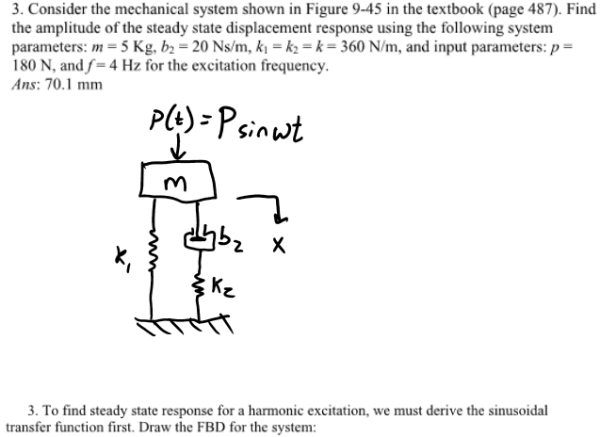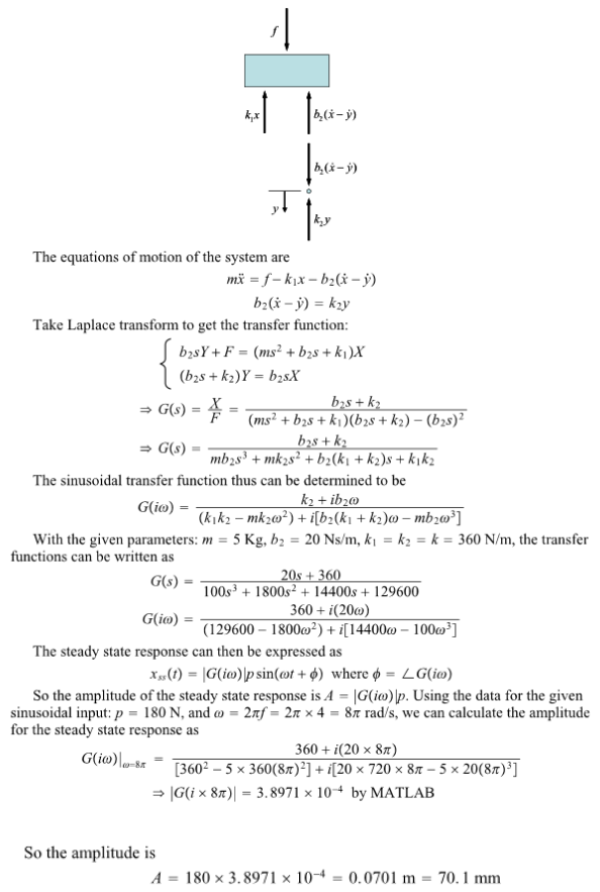Consider the mechanical system shown in the figure in the textbook. Find the amplitude of the steady state displacement response using the following system parameters: m=5 kg, b2= 20 N/sm, k1=k2=k=360 n/m, and input parameters: p=180 N, and f=4 Kz for the excitation frequency.Consider the mechanical system shown in the figure in the textbook. Find the amplitude of the steady state displacement response using the following system parameters: m=5 kg, b2= 20 N/sm, k1=k2=k=360 n/m, and input parameters: p=180 N, and f=4 Kz for the excitation frequency.

System Dynamics Page 2 dynamics dynamics dynamics dynamics dynamics dynamics dynamics System dynamics Page 3